Next: Decomposition of Tide Generating Up: Terrestrial Ocean Tides Previous: Introduction

# Tide Generating Potential

Consider an (almost) spherical planet of mass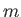, and radius, and a spherical moon of mass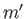, that execute (almost) circular orbits about their mutual center of mass. Let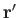(where) represent the vector position of the center of the moon, relative to the center of the planet. It follows that (Fitzpatrick 2012)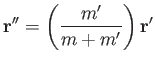(12.1)

is the vector position of the center of mass, relative to the center of the planet. Moreover, the planet and moon both orbit the center of mass with angular velocity, where (Fitzpatrick 2012)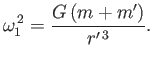(12.2)

Here,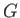is the universal gravitational constant (Yoder 1995).

Neglecting the planet's axial rotation (which is introduced into our analysis in Section 12.6), the planet's orbital motion about the center of mass causes its constituent points to execute circular orbits of radius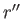with angular velocity. The centers of these orbits have the same spatial relation to the center of mass that the constituent points have to the center of the planet. Moreover, the orbits lie in parallel planes. Thus, the common centripetal acceleration of the constituent points is (Fitzpatrick 2012)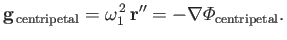(12.3)

It follows, from the previous three equations, that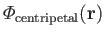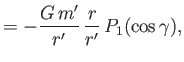(12.4)

where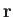is a position vector relative to the center of the planet, and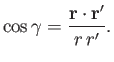(12.5)

Here,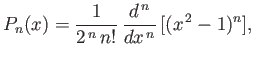(12.6)

for, denotes a Legendre polynomial (Abramowitz and Stegun 1965). In particular,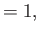(12.7)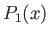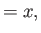(12.8)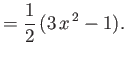(12.9)

The gravitational force per unit mass exerted by the moon on one of the planet's constituent points, located at position vector, is written(12.10)

where (Fitzpatrick 2012)(12.11)

assuming that. The tidal force per unit mass acting on the point (i.e., the force per unit mass that is not compensated by the centripetal acceleration) is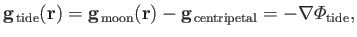(12.12)

where(12.13)

is termed the tide generating potential. Thus, according to Equations (12.4) and (12.11),(12.14)

The truncation of the expansion is ultimately justified by the fact that.Next: Decomposition of Tide Generating Up: Terrestrial Ocean Tides Previous: Introduction
Richard Fitzpatrick 2016-03-31